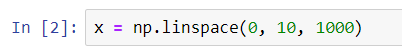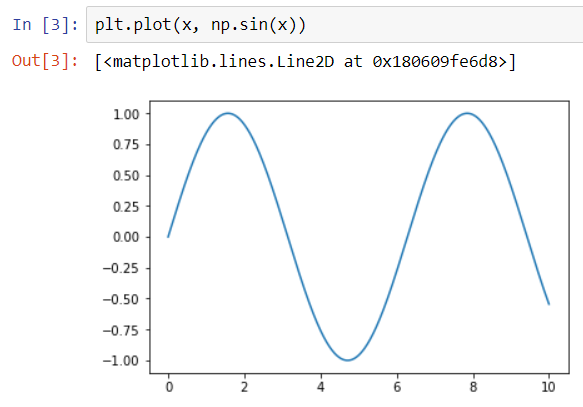A simple line plot can be a straight line, simple curve, trigonometric functions, etc.

Let us suppose that we want to plot a sinusoidal curve in Matplotlib.

We will first import the required Matplotlib and NumPy libraries.Next, we will create a set of values for our sinusoid function to take as input.Here, x contains a set of 1000 values ranging from 0 to 10.

Finally, we can plot out sinusoid as follows -